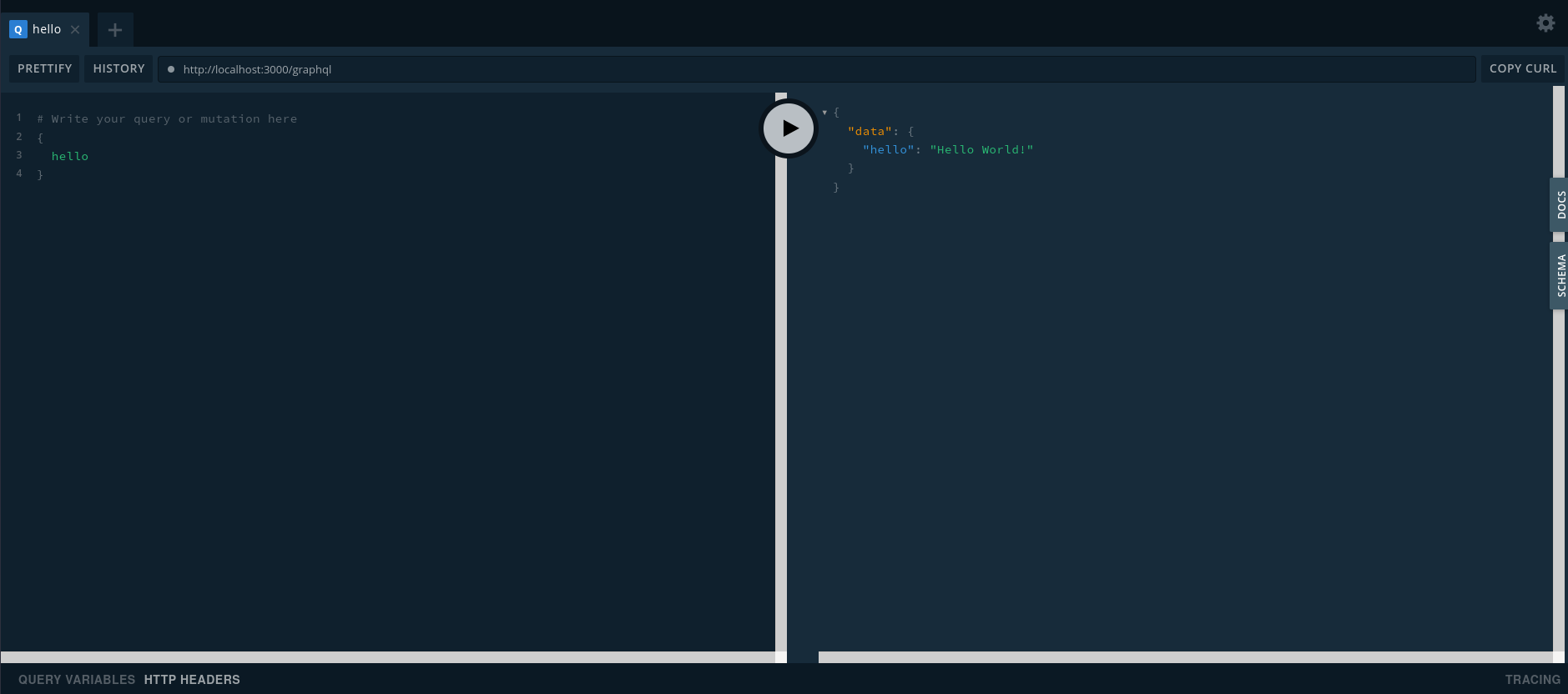# Building a GraphQL API with Deno and gql

In this post I would like to show how to build a GraphQL API server with gql. At the moment there are a few GraphQL server modules for Deno, such as obsidian and oak_graphql but all of them are either standalone or framework-specific. gql instead, is a framework-agnostic middleware so I will use it with Deno std's `http`.

## .css-1h1ulo5{position:absolute;left:-2rem;-webkit-text-decoration:none;text-decoration:none;color:var(--fg-dark);}.css-1h1ulo5:hover{color:var(--fg-hover);}#GraphQL Schema

We'll start with declaring a schema using type definitions and resolvers using graphql_tag and graphql_tools Deno modules, similar to how you do it with Apollo Server or GraphQL Yoga:

```.css-1sgc73c{display:-webkit-box;display:-webkit-flex;display:-ms-flexbox;display:flex;-webkit-flex-direction:column;-ms-flex-direction:column;flex-direction:column;font-size:14px;}.css-1kklwad{border:none;background:transparent;color:var(--fg-dark);display:inherit;-webkit-box-pack:center;-ms-flex-pack:center;-webkit-justify-content:center;justify-content:center;width:-webkit-max-content;width:-moz-max-content;width:max-content;-webkit-align-items:center;-webkit-box-align:center;-ms-flex-align:center;align-items:center;-webkit-align-self:flex-end;-ms-flex-item-align:flex-end;align-self:flex-end;padding:0;margin:0;}.css-1kklwad:hover{cursor:pointer;}.css-1kklwad img{margin-left:10px;}.css-1wxf3li{border:1px solid var(--fg-dark);padding:1rem;overflow-x:auto;}@media (max-width: 980px){.css-1wxf3li{overflow-x:scroll;}}`import`` ``{`` makeExecutableSchema ``}`` ``from`` ``'https://deno.land/x/graphql_tools/mod.ts'``import`` ``{`` gql ``}`` ``from`` ``'https://deno.land/x/graphql_tag/mod.ts'````
````/* Type definitions */``const`` typeDefs ``=`` gql`````  ``type`` ``Query`` ``{``    ``hello``:`` ``String``  ``}```````
````/* Resolvers */``const`` resolvers ``=`` ``{``  Query``:`` ``{``    ``hello``:`` ``(``)`` ``=>`` `````Hello World!`````  ``}``}````
````const`` schema ``=`` ``makeExecutableSchema``(``{`` resolvers``,`` typeDefs ``}``)````

Now we have an executable schema that can be passed to a GraphQL server.

## #Server setup

In order to setup gql you just need to pass a `req` object to it so it can read request body and properties.

````import`` ``{`` serve ``}`` ``from`` ``'https://deno.land/std@0.90.0/http/server.ts'``import`` ``{`` GraphQLHTTP ``}`` ``from`` ``'https://deno.land/x/gql/mod.ts'``import`` ``{`` makeExecutableSchema ``}`` ``from`` ``'https://deno.land/x/graphql_tools/mod.ts'``import`` ``{`` gql ``}`` ``from`` ``'https://deno.land/x/graphql_tag/mod.ts'````
````const`` typeDefs ``=`` gql`````  ``type`` ``Query`` ``{``    ``hello``:`` ``String``  ``}```````
````const`` resolvers ``=`` ``{``  Query``:`` ``{``    ``hello``:`` ``(``)`` ``=>`` `````Hello World!`````  ``}``}````
````const`` schema ``=`` ``makeExecutableSchema``(``{`` resolvers``,`` typeDefs ``}``)````
````const`` s ``=`` ``serve``(``{`` port``:`` ``3000`` ``}``)````
````for`` ``await`` ``(``const`` req ``of`` s``)`` ``{``  req``.``url``.``startsWith``(``'/graphql'``)``    ``?`` ``await`` ``GraphQLHTTP``(``{``        schema``,``        graphiql``:`` ``true`` ``// enable GraphQL playground``      ``}``)``(``req``)``    ``:`` req``.``respond``(``{``        status``:`` ``404``      ``}``)````

Now run the server with these permissions (for reading body and using network):

``deno run --allow-net --allow-read server.ts``

## #GraphQL Playground

Now when then server is up, the GraphQL Playground will launch as well.

Open your favourite browser on http://localhost:3000 and you will see this:## #Server Context

It's also possible to pass request context to a schema so it could be used in resolvers. Request object is automatically passed to the context function.

````import`` ``{`` serve``,`` ServerRequest ``}`` ``from`` ``'https://deno.land/std@0.90.0/http/server.ts'``import`` ``{`` GraphQLHTTP ``}`` ``from`` ``'https://deno.land/x/gql/mod.ts'``import`` ``{`` makeExecutableSchema ``}`` ``from`` ``'https://deno.land/x/graphql_tools/mod.ts'``import`` ``{`` gql ``}`` ``from`` ``'https://deno.land/x/graphql_tag/mod.ts'````
````const`` typeDefs ``=`` gql`````  ``type`` ``Query`` ``{``    ``hello``:`` ``String``  ``}```````
````const`` resolvers ``=`` ``{``  Query``:`` ``{``    ``hello``:`` ``(``root``,`` args``,`` ctx``)`` ``=>`` `````Hello World from ``\${``ctx``.``url``}``! Cusotm context property ``\${``ctx``.``ctxProp``}`````  ``}``}````
````const`` schema ``=`` ``makeExecutableSchema``(``{`` resolvers``,`` typeDefs ``}``)````
````const`` s ``=`` ``serve``(``{`` port``:`` ``3000`` ``}``)````
````for`` ``await`` ``(``const`` req ``of`` s``)`` ``{``  req``.``url``.``startsWith``(``'/graphql'``)``    ``?`` ``await`` ``GraphQLHTTP``<``ServerRequest``>``(``{``        schema``,``        graphiql``:`` ``true`` ``// enable GraphQL playground,``        ``context``:`` ``(``request``)`` ``=>`` ``(``{``          request``,`` ``// request object``          ctxProp``:`` ``'ctxValue'`` ``// aditional context properties``        ``}``)``      ``}``)``(``req``)``    ``:`` req``.``respond``(``{``        status``:`` ``404``      ``}``)````

## #Conclusion

This is how you can set up a simple GraphQL server for Deno without any backend frameworks, just `std/http`. Although, there are a few examples of using gql with other frameworks, check them out.